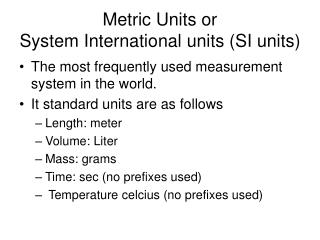DownloadDownload PresentationMetric Units or System International units (SI units)

Metric Units or System International units (SI units)

Download PresentationMetric Units or System International units (SI units)

- - - - - - - - - - - - - - - - - - - - - - - - - - - E N D - - - - - - - - - - - - - - - - - - - - - - - - - - -
Presentation Transcript

1. Metric Units or System International units (SI units) • The most frequently used measurement system in the world. • It standard units are as follows • Length: meter • Volume: Liter • Mass: grams • Time: sec (no prefixes used) • Temperature celcius (no prefixes used)

2. Metric Conversions Measure the lengths of the following items located in the classroom using centimeters. Record your findings • Your desk • Text Book • Fish tank Height • Classroom door • Pencil or pen • An item of your choice

3. The Conversion Challenge!

4. Write the correct abbreviation Kilogram: Milliliter: Gram: Millimeter: Kilometer: Write the correct abbreviation Kilogram: Milliliter: Gram: Millimeter: Kilometer:

5. Write the correct abbreviation Liter: Centimeter: Milligram: meter: Write the correct abbreviation Liter: Centimeter: Milligram: meter:

6. 400mg = ______ g 15 km = ______ m 400mg = ______ g 15 km = ______ m

7. 823ml = _______L 82 g = ________mg 823ml = _______L 82 g = ________mg

8. Compare using <, > or = Compare using <, > or = 72 cm 7m 543 cm 54.3mm 72 cm 7m 543 cm 54.3mm

9. Compare using <, > or = Compare using <, > or = 6g 610 mg 543 cm 54.3decimeters 6g 610 mg 543 cm 54.3decimeter

10. 4.3 m = _______cm 4.3 m = _______cm 4kg = ______mg 4kg = ______mg

11. 130 mg = ______kg 604 mm = ______ m 130 mg = ______kg 604 mm = ______ m

12. Compare using <, > or = 1200mL 1.2L 483Kg 4830mg Compare using <, > or = 1200mL 1.2L 483Kg 4830mg

13. Compare using <, > or = 12000mL 1.2L 483g 4830mg Compare using <, > or = 1200mL 1.2L 483Kg 4830mg

14. 54kg = _________mg 676 mL = _________ L 5400kg = _________mg 676 mL = _________ L

15. 3 Km = _______ cm 375 decigrams = _______Kg 3456 Km = _______ cm 375 decigrams = _______Kg

16. 1676 hectaliter = _______ L 32 g = _______ centigrams 1676 hectoliter = _______ L 32 g = _______ centigrams

17. 7003 Dekagram = ______g 19 Kiloliter = _________Deciliter 7003 Dekagram = ______g 190 Kiloliter = _________Deciliter

18. English Measurements into Metric • 1 litter = 4.2 cups • 1 Km = .62 miles • 1 pound = 1.45 kg • 1 in = 2.54 cm • 1 foot = 30.48 cm • 1 cup = 240 ml